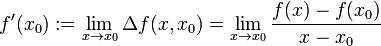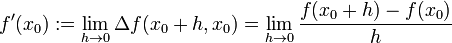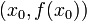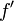# Derivative

## Definition at a point

### Algebraic definition

Suppose$f$ is a function defined on a subset of the reals and$x_0$ is a point in the interior of the domain of$f$, i.e., the domain of$f$ contains an open interval surrounding$x_0$. The derivative (also called first derivative) of$f$ at$x_0$, denoted$f'(x_0)$ is defined as the limit of the difference quotient of$f$ between$x_0$ and$x$, as$x \to x_0$. Explicitly:$\! f'(x_0) := \lim_{x \to x_0} \Delta f(x,x_0) = \lim_{x \to x_0} \frac{f(x) - f(x_0)}{x - x_0}$

If this limit exists, then we say that the derivative exists and has this value, and we say that the function is differentiable at the point. If the limit does not exist, then we say that the function is not differentiable at the point and the derivative does not exist.

### Computationally useful version of algebraic definition

This is obtained from the previous definition by the variable substitution$h := x - x_0$ so$x = x_0 + h$. Explicitly:$\! f'(x_0) := \lim_{h \to 0} \Delta f(x_0 + h,x_0) = \lim_{h \to 0} \frac{f(x_0 + h) - f(x_0)}{h}$

### Geometric definition

Suppose$f$ is a function and$x_0$ is a point in the interior of the domain of$f$, i.e., the domain of$f$ contains an open interval surrounding$x_0$. The derivative of$f$ at$x_0$ is the slope of the tangent line to the graph of$f$ through the point$(x_0,f(x_0))$.

## Definition as a function

Suppose$f$ is a function defined on a subset of the reals. Its derivative or first derivative, denoted$\! f'$, is a function defined as follows:

• The domain is the following subset of the domain of$f$: An element in the domain of$f$ is in the domain of$f'$ if and only if it is in the interior of the domain of$f$ and the derivative of$f$ exists at the point.
• The function value at any point in the domain is simply the value of the derivative of$f$ at that point.
MORE ON THE WAY THIS DEFINITION OR FACT IS PRESENTED: We first present the version that deals with a specific point (typically with a$\{ \}_0$ subscript) in the domain of the relevant functions, and then discuss the version that deals with a point that is free to move in the domain, by dropping the subscript. Why do we do this?
The purpose of the specific point version is to emphasize that the point is fixed for the duration of the definition, i.e., it does not move around while we are defining the construct or applying the fact. However, the definition or fact applies not just for a single point but for all points satisfying certain criteria, and thus we can get further interesting perspectives on it by varying the point we are considering. This is the purpose of the second, generic point version.

## Leibniz notation for derivative

Fill this in later